Areas Related to Circles

# Class 10 Math Chapter 11 Areas Related To Circles Important QuestionsHere are some important questions for Class 10 Mathematics Chapter 11, Areas Related to Circles, carefully selected to help students prepare effectively for the CBSE Class 10 Mathematics Examination in 2023-24. By practicing these varied problems, students can better understand the concepts of Areas Related to Circles and improve their problem-solving skills. These questions aim to clarify doubts and enhance performance in the chapter.

## Introduction

In Chapter 11 of Class 10 Mathematics, Areas Related To Circles, we explore concepts related to the Area of Sectors and Segments of a circle. The problems in this chapter focus on calculating the areas and perimeters (or circumferences) of these plane figures. When calculating the area of a segment of a circle, the problems are restricted to central angles of 60°, 90°, and 120°. This chapter delves into understanding how to find the areas of different portions of a circle, as well as solving problems that involve both areas and perimeters/circumferences.

### What is the area of a sector of a circle?

The area of a sector of a circle is the region enclosed by an arc and two radii of the circle. It is calculated using the formula,
A = (θ/360) × πr², where 'θ' is the central angle and 'r' is the radius.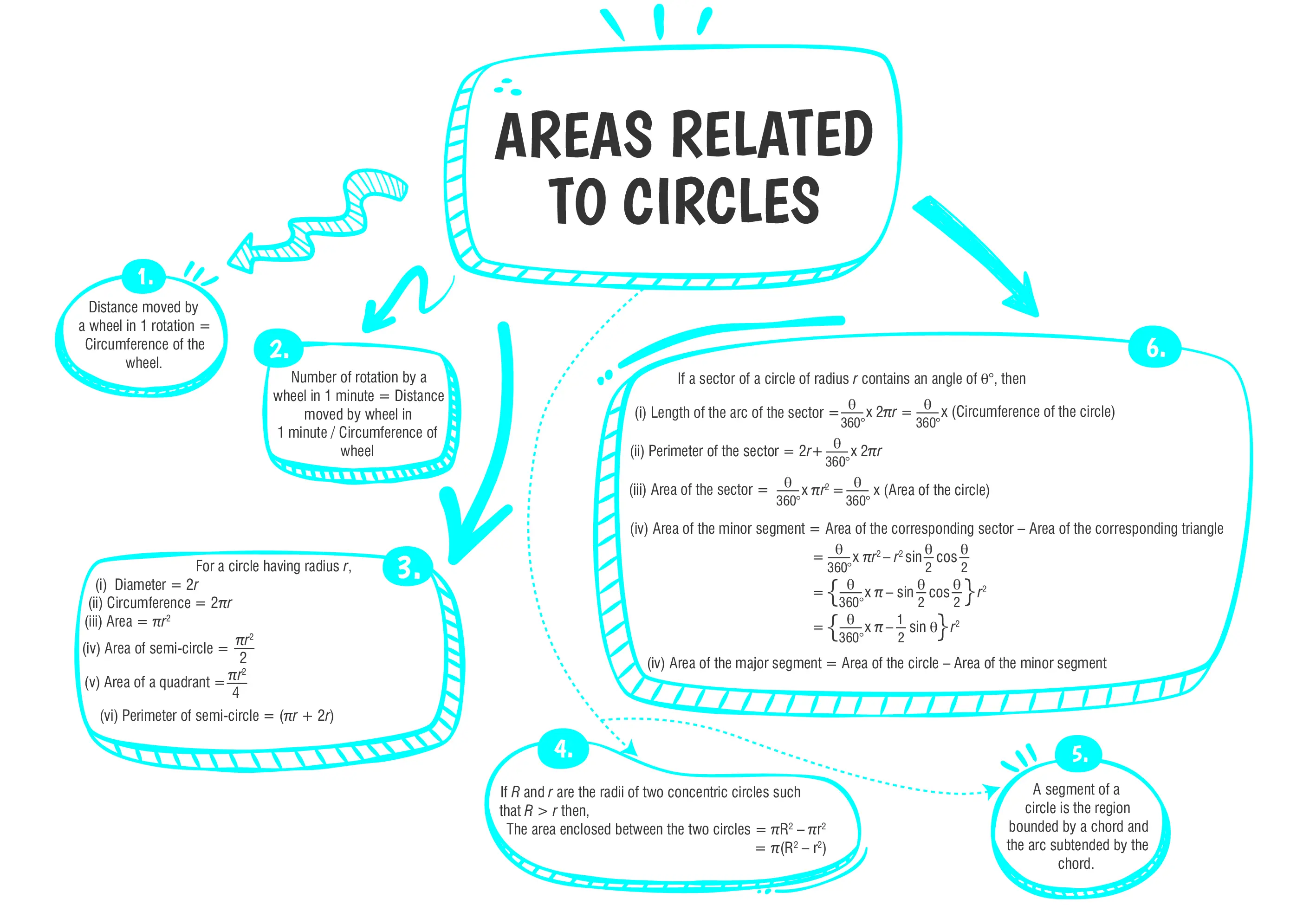### Class 10 Areas Related To Circles Important Questions and Answers

#### Q1.  In the given figure, if ∠AOD = 135°, then ∠BOC is equal to: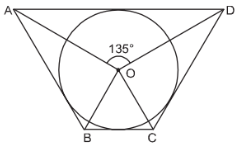#### (a) 52.5°(b) 45°(c) 62.5°(d) 25°

Ans. (b) 45°

Explanation:
Given, ∠AOD = 135°
∴ ∠BOC = 180° – 135° = 45°
Since, angles subtended at the center by a pair of opposite sides are supplementary.

#### Options

(a)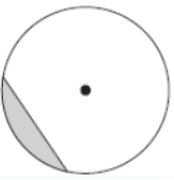(b)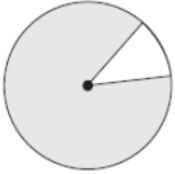(c)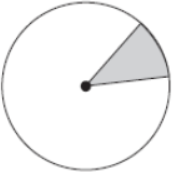(d)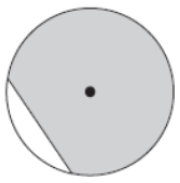Ans. (b)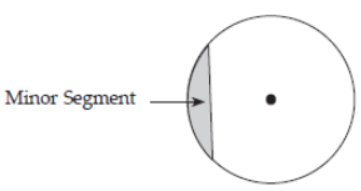Explanation:
The shaded region consists of a chord and the arcs of the circle. The region in the white has the major arc, so it is the major segment and the shaded  region consists of the minor arc, so it is the minor segment.

#### Q3. From each of the two adjacent corners of a square of side 8 cm, a quadrant of a circle of radius 1.4 cm is cut. Another circle of radius 4.2 cm is also cut from the center as shown in the given figure. Find the area of the remaining portion of the square.$$Use π =\frac{22}{7}$$

Ans. Area = 5.48 cm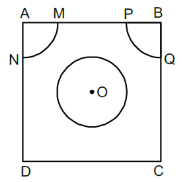Explanation:
Given, AB = BC = CD = DA = 8 cm,
AM = AN = PB = QB = 1.4 cm and radius of circle with center O = 4.2 cm
Thus, area of the remaining portion
= Area of ABCD
- [Area of circle + 2 Area of quadrants]
=8×8- $$\begin{Bmatrix} \frac{22}{7}(4.2)^2 +2 ×\frac{1}{4}×\frac{22}{7}(1.4)^2 \end{Bmatrix}$$
= 64 - (55.44 + 3.08) cm2
= 5.48 cm2

#### Q4. The length of the minute hand of a clock is 14 cm. Find the area swept by the minute hand in 5 minutes.

Ans. Area swept by the minute hand in covering 5 minutes = 51.33 cm2

Explanation:
Given, length of the minute hand = r = 14 cm Total number of divisions in the clock = 12 Hence the angle subtended at the center between two digits covering five minutes
$$=\frac{360\degree}{12}=30\degree$$
Hence, area swept by the minute hand in covering 5 minutes
$$=\frac{\theta}{360\degree}$$ $$\pi^2$$
$$=\frac{30\degree}{360\degree}×\frac{22}{7}×(14)^2$$
$$=\frac{154}{3}$$
= 51.33 cm2.

#### Q5.  From a thin metallic piece in the shape of a trapezium ABCD in which AB || CD and ∠BCD = 90°, a quarter circle BFEC is removed as shown in the figure. Given, AB = BC = 3.5 cm and DE = 2 cm, calculate the area of the remaining part of the metal sheet.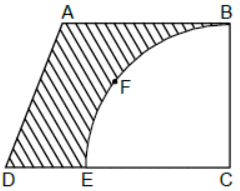Ans. Area of the remaining part of the metal sheet = 6.125 cm2

Explanation:
Given, AB =BC =3.5 cm and DE = 2 cm
As BC and EC are the radii of the circle, so
BC = EC
Thus, DC = (3.5+2) cm
= 5.5 cm
Hence , area  of remaining part of the metal sheet
= Area of the trapezium - Area of the quadrant
$$=\frac{1}{2}× \text{sum of parallel sides}$$
× $$\text{distance between parallel sides} -\frac{1}{4}\pi r^2$$
$$=\frac{1}{2}×(3.5 + 5.5) × 3.5$$- $$\frac{1}{4}×\frac{22}{7}×(3.5)^2$$
$$=\frac{1}{2}× 9 × 3.5-\frac{11×0.5×3.5}{2}$$
$$=\frac{31.5}{2}-\frac{19.25}{2}$$
$$=\frac{12.25}{2}$$
$$= 6.125 cm^2$$#### CBSE Class 10 Maths Chapter wise Important Questions

Chapter No. Chapter Name
Chapter 1 Real Number
Chapter 2 Polynomials
Chapter 3 Pair of Linear Equations in Two Variables
Chapter 5 Arithmetic Progressions
Chapter 6 Triangles
Chapter 7 Coordinate Geometry
Chapter 8 Introduction to Trigonometry
Chapter 9 Some Applications of Trigonometry
Chapter 10 Circles
Chapter 11 Areas Related to Circle
Chapter 12 Surface Areas and Volumes
Chapter 13 Statistics
Chapter 14 Probability

#### Conclusion

To enhance your understanding of the Areas Related to Circles chapter, consider exploring oswal.io. This website provides a variety of practice questions designed to facilitate effective learning. By working on these questions, you can improve your grasp of circle-related concepts and enhance your problem-solving skills in this crucial math topic.

#### Q1: What is a segment of a circle?

Ans: A segment of a circle is the region bounded by an arc and a chord. It is like a "slice" of the circle. There are minor and major segments.

#### Q2: What are some practical applications of the areas related to circle?

Ans: The area of a circle is used in various real-life scenarios, such as calculating the area of circular fields, pools, circular objects, and designing circular structures.

#### Q3: How is the perimeter or circumference of a sector related to its area?

Ans: The perimeter (circumference) of a sector consists of the arc's length and two radii. The area of the sector includes the region enclosed by the arc and radii.

#### Q4: How does the formula for the area of a segment change for a major segment compared to a minor segment?

Ans: For a major segment, subtract the area of a triangle from the area of a sector, while for a minor segment, add the area of a triangle to the area of a sector.

#### Q5: What is the relation between the central angle of a circle and the area of the corresponding sector?

Ans: The area of a sector is directly proportional to the measure of its central angle. If two sectors have the same central angle, their areas will be proportional to the squares of their radii.

###### Copyright 2022 OSWAL PUBLISHERS Simplifying ExamsPhone:  (+91) 78959 87722
Mail: support@oswalpublishers.in